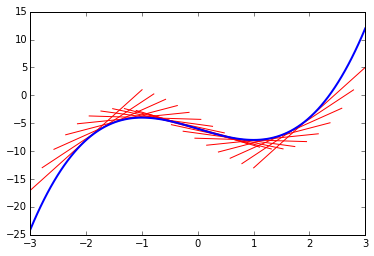# 不定积分

## 常微分方程（Ordinary Differential Equations, ODE）t = sympy.Symbol('t')
c = sympy.Symbol('c')
domain = np.linspace(-3,3,100)
v = t**3-3*t-6
a = v.diff()

for p in np.linspace(-2,2,20):
slope = a.subs(t,p)
intercept = sympy.solve(slope*p+c-v.subs(t,p),c)
lindomain = np.linspace(p-1,p+1,20)
plt.plot(lindomain,slope*lindomain+intercept,'red',linewidth = 1)

plt.plot(domain,[v.subs(t,i) for i in domain],linewidth = 2)## 不定积分（Indefinite Integral）print a.integrate()
# result is : t**3 - 3*t
print sympy.integrate(sympy.E**t+3*t**2)
# result is : t**3 + exp(t)